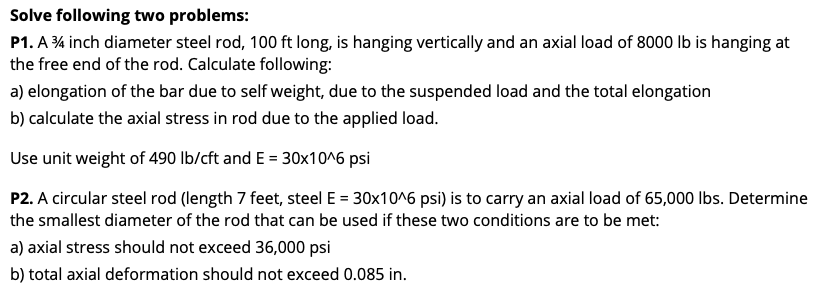# Question Solve following two problems: P1. A 34 inch diameter steel rod, 100 ft long, is hanging vertically and an axial load of 8000 lb is hanging at the free end of the rod. Calculate following: a) elongation of the bar due to self weight, due to the suspended load and the total elongation b) calculate the axial stress in rod due to the applied load. Use unit weight of 490 lb/cft and E = 30x10^6 psi P2. A circular steel rod (length 7 feet, steel E = 30x10^6 psi) is to carry an axial load of 65,000 lbs. Determine the smallest diameter of the rod that can be used if these two conditions are to be met: a) axial stress should not exceed 36,000 psi b) total axial deformation should not exceed 0.085 in.